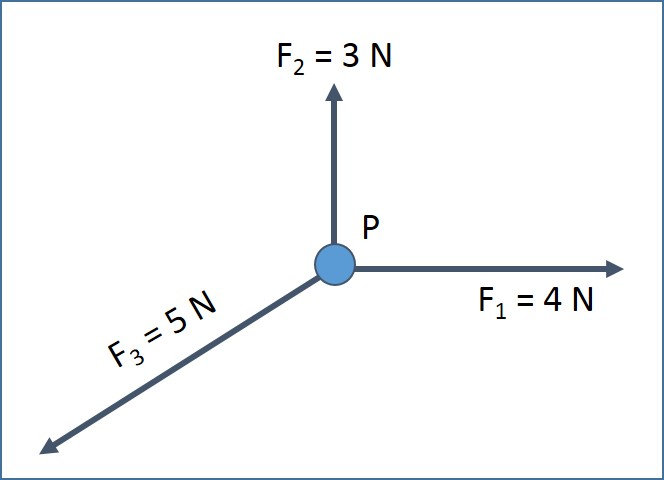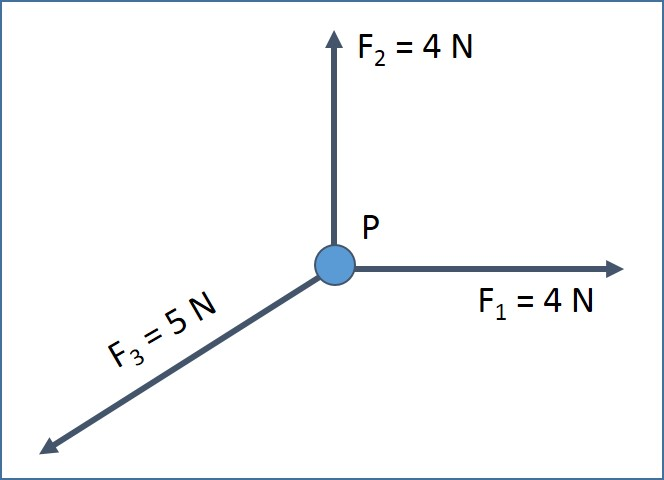# Conditions For Equilibrium

## Introduction

An object is said to be in equilibrium if the resultant force acting on the object is zero or the sum of the moments acting on the object is zero. This article discusses the methods to find out force equilibrium.

A. Resolving Vectors

In this method, all the vectors acting on an object are resolved into horizontal and vector components. If the sum of all the horizontal components and sum of all the vertical components are zero, then the forces acting on the object are in equilibrium. The following equations shall be used to resolve the force vector.B. Closed Triangle

When all the forces acting on an object are drawn to scale, if a closed triangle is formed, then the forces are said to be in equilibrium. Figure 1 illustrates three forces plotted with quantity and direction. Figure 2 shows that a closed triangle is formed when these three forces are plotted. Therefore, forces F1, F2 and F3 acting on object P are in equilibrium.Figure 1: Forces F1, F2 and F3Figure 2: Closed Triangle Formed by Forces F1, F2 and F3

Figure 3 illustrates another three forces plotted with quantity and direction. Figure 4 shows that a closed triangle is not formed when these three forces are plotted. Therefore, forces F1, F2 and F3 acting on object P are not in equilibrium.Figure 3: Forces F1, F2 and F3Figure 4: Forces Not in Equilibrium

C. Summary

• An object is said to be in equilibrium if the resultant force acting on the object is zero or the sum of the moments acting on the object is zero.
• If the sum of all the horizontal components and sum of all the vertical components are zero, then the forces acting on the object are in equilibrium
• When all the forces acting on an object are drawn to scale, if a closed triangle is formed, then the forces are said to be in equilibrium.